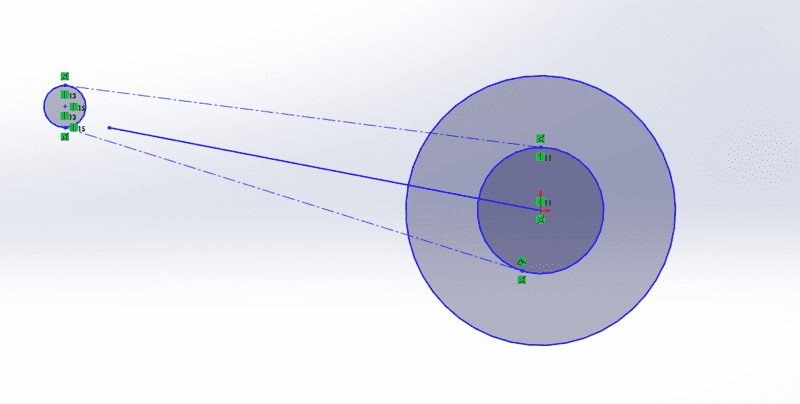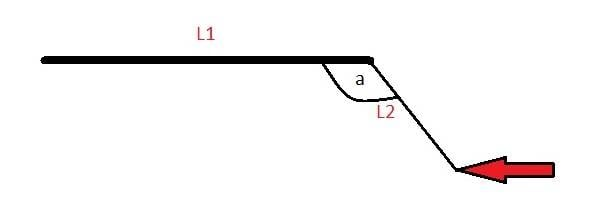# Calculating torque at a distance from the lever

• Ross Hanna
In summary, the speaker is an engineering student who is struggling with calculating the overall moments of a motorcycle's rear end. They have no problem with the first two moments, but are struggling with the third. They have provided a diagram and an equation they found through research, but they do not fully understand how it works. They are seeking help in understanding how to calculate the torque in this system.

#### Ross Hanna

Hi there, I am an engineering student studying my masters, but have run into a roadblock in my calculations. I know I'm missing something obvious but i just can't see it. Too close to the woods and all that!

Anyway, i have the rear end of a motorcycle. to calculatew the overall moments, i have to find the three individual moments acting on it. the first two I'm fine with, but the third I'm struggling.

I have a rear end of a motorcycle. The wheel has a sprocket/ gear rigidly attached. A chain connects this gear to another at a distance Lc centre to centre and angle eta with a tension T. Lc is constructed of a triangle with sides a (horizontal) and b (vertical). The wheel is attached to a swingarm with length L, and angle to the horizontal of phi. The gears have radius Rc and Rs. The front sprocket rotates at V rad/s and transmits a power P.

I have attached a diagram to help.From research, the moment should be;

T*(Rc - L*sin(phi-eta))

I can get the equation by working backwards, but i don't understand how it works. The basics physics question is this. If i have a system such as below, how do i calculate the torque?if the torque was applid to L1, i would just use T = F.r.sin(theta), but I'm unsure how to resolve the forces for this.

Thanks for any help

Ross

#### Attachments

When you say you're trying to calculate "the overall moments" what are you referring to?

I'm kind of assuming the speed/acceleration of the driving wheel, in which case the suspension geometry doesn't play into the calculation at all. Just compare the ratio or radii between the two sprockets for the torque multiplication, and apply a reasonable efficiency factor for power loss through the chain drive.

Am I missing something that you're trying to calculate?